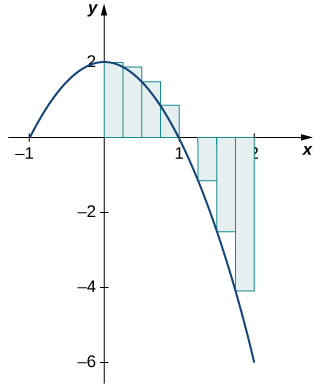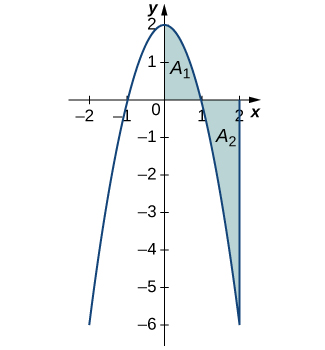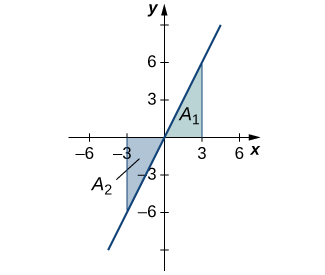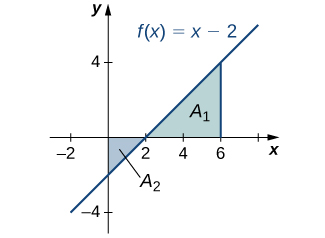# 5.2 The definite integral  (Page 3/16)

 Page 3 / 16

Use the formula for the area of a trapezoid to evaluate ${\int }_{2}^{4}\left(2x+3\right)dx.$

18 square units

## Area and the definite integral

When we defined the definite integral, we lifted the requirement that $f\left(x\right)$ be nonnegative. But how do we interpret “the area under the curve” when $f\left(x\right)$ is negative?

## Net signed area

Let us return to the Riemann sum. Consider, for example, the function $f\left(x\right)=2-2{x}^{2}$ (shown in [link] ) on the interval $\left[0,2\right].$ Use $n=8$ and choose $\left\{{x}_{i}^{*}\text{}}$ as the left endpoint of each interval. Construct a rectangle on each subinterval of height $f\left({x}_{i}^{*}\right)$ and width Δ x . When $f\left({x}_{i}^{*}\right)$ is positive, the product $f\left({x}_{i}^{*}\right)\text{Δ}x$ represents the area of the rectangle, as before. When $f\left({x}_{i}^{*}\right)$ is negative, however, the product $f\left({x}_{i}^{*}\right)\text{Δ}x$ represents the negative of the area of the rectangle. The Riemann sum then becomes

$\sum _{i=1}^{8}f\left({x}_{i}^{*}\right)\text{Δ}x=\left(\text{Area of rectangles above the}\phantom{\rule{0.2em}{0ex}}x\text{-axis}\right)-\left(\text{Area of rectangles below the}\phantom{\rule{0.2em}{0ex}}x\text{-axis}\right)$For a function that is partly negative, the Riemann sum is the area of the rectangles above the x -axis less the area of the rectangles below the x -axis.

Taking the limit as $n\to \infty ,$ the Riemann sum approaches the area between the curve above the x -axis and the x -axis, less the area between the curve below the x -axis and the x -axis, as shown in [link] . Then,

$\begin{array}{cc}{\int }_{0}^{2}f\left(x\right)dx\hfill & =\underset{n\to \infty }{\text{lim}}\sum _{i=1}^{n}f\left({c}_{i}\right)\text{Δ}x\hfill \\ & ={A}_{1}-{A}_{2}.\hfill \end{array}$

The quantity ${A}_{1}-{A}_{2}$ is called the net signed area    .In the limit, the definite integral equals area A 1 less area A 2 , or the net signed area.

Notice that net signed area can be positive, negative, or zero. If the area above the x -axis is larger, the net signed area is positive. If the area below the x -axis is larger, the net signed area is negative. If the areas above and below the x -axis are equal, the net signed area is zero.

## Finding the net signed area

Find the net signed area between the curve of the function $f\left(x\right)=2x$ and the x -axis over the interval $\left[-3,3\right].$

The function produces a straight line that forms two triangles: one from $x=-3$ to $x=0$ and the other from $x=0$ to $x=3$ ( [link] ). Using the geometric formula for the area of a triangle, $A=\frac{1}{2}bh,$ the area of triangle A 1 , above the axis, is

${A}_{1}=\frac{1}{2}3\left(6\right)=9,$

where 3 is the base and $2\left(3\right)=6$ is the height. The area of triangle A 2 , below the axis, is

${A}_{2}=\frac{1}{2}\left(3\right)\left(6\right)=9,$

where 3 is the base and 6 is the height. Thus, the net area is

${\int }_{-3}^{3}2xdx={A}_{1}-{A}_{2}=9-9=0.$The area above the curve and below the x -axis equals the area below the curve and above the x -axis.

Analysis

If A 1 is the area above the x -axis and A 2 is the area below the x -axis, then the net area is ${A}_{1}-{A}_{2}.$ Since the areas of the two triangles are equal, the net area is zero.

Find the net signed area of $f\left(x\right)=x-2$ over the interval $\left[0,6\right],$ illustrated in the following image.6

## Total area

One application of the definite integral is finding displacement when given a velocity function. If $v\left(t\right)$ represents the velocity of an object as a function of time, then the area under the curve tells us how far the object is from its original position. This is a very important application of the definite integral, and we examine it in more detail later in the chapter. For now, we’re just going to look at some basics to get a feel for how this works by studying constant velocities.

how does this work
Can calculus give the answers as same as other methods give in basic classes while solving the numericals?
log tan (x/4+x/2)
Rohan
Rohan
y=(x^2 + 3x).(eipix)
Claudia
Ismael
A Function F(X)=Sinx+cosx is odd or even?
neither
David
Neither
Lovuyiso
f(x)=1/1+x^2 |=[-3,1]
apa itu?
fauzi
determine the area of the region enclosed by x²+y=1,2x-y+4=0
Hi
MP
Hi too
Vic
hello please anyone with calculus PDF should share
Which kind of pdf do you want bro?
Aftab
hi
Abdul
can I get calculus in pdf
Abdul
How to use it to slove fraction
Hello please can someone tell me the meaning of this group all about, yes I know is calculus group but yet nothing is showing up
Shodipo
You have downloaded the aplication Calculus Volume 1, tackling about lessons for (mostly) college freshmen, Calculus 1: Differential, and this group I think aims to let concerns and questions from students who want to clarify something about the subject. Well, this is what I guess so.
Jean
Im not in college but this will still help
nothing
how can we scatch a parabola graph
Ok
Endalkachew
how can I solve differentiation?
with the help of different formulas and Rules. we use formulas according to given condition or according to questions
CALCULUS
For example any questions...
CALCULUS
v=(x,y) وu=(x,y ) ∂u/∂x* ∂x/∂u +∂v/∂x*∂x/∂v=1
log tan (x/4+x/2)
Rohan
what is the procedures in solving number 1?
review of funtion role?
for the function f(x)={x^2-7x+104 x<=7 7x+55 x>7' does limx7 f(x) exist?
find dy÷dx (y^2+2 sec)^2=4(x+1)^2
Integral of e^x/(1+e^2x)tan^-1 (e^x)
why might we use the shell method instead of slicing
fg[[(45)]]²+45⅓x²=100By Richley CrapoBy OpenStaxBy OpenStaxBy Marion CabalfinBy Janet ForresterBy Madison ChristianBy Abishek DevarajBy JavaChamp TeamBy Eddie UnverzagtBy Jazzycazz Jackson